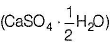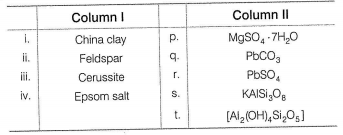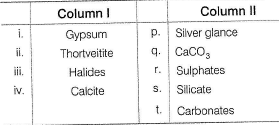Courses

# Test: Introduction To Metallurgy And Ores Of Some Metals

## 25 Questions MCQ Test Chemistry Class 12 | Test: Introduction To Metallurgy And Ores Of Some Metals

Description
This mock test of Test: Introduction To Metallurgy And Ores Of Some Metals for Class 12 helps you for every Class 12 entrance exam. This contains 25 Multiple Choice Questions for Class 12 Test: Introduction To Metallurgy And Ores Of Some Metals (mcq) to study with solutions a complete question bank. The solved questions answers in this Test: Introduction To Metallurgy And Ores Of Some Metals quiz give you a good mix of easy questions and tough questions. Class 12 students definitely take this Test: Introduction To Metallurgy And Ores Of Some Metals exercise for a better result in the exam. You can find other Test: Introduction To Metallurgy And Ores Of Some Metals extra questions, long questions & short questions for Class 12 on EduRev as well by searching above.
QUESTION: 1

### Only One Option Correct Type This section contains 10 multiple choice questions. Each question has four choices (a), (b), (c) and (d), out of which ONLY ONE is correct Q. Which represents the correct abundance order of elements in the earth crust?

Solution:

The mass-abundance of the nine most abundant elements in the Earth's crust is approximately: oxygen 46%, silicon 28%, aluminum 8.3%, iron 5.6%, calcium 4.2%, sodium 2.5%, magnesium 2.4%, potassium 2.0%, and titanium 0.61%.

QUESTION: 2

### Which of the following contains both copper and iron?

Solution:

Chalcopyrite (CuFeS2) is the ore which contains both copper and iron.

QUESTION: 3

### Regarding cryolite incorrect statement is

Solution:

Cryolite (Na3AIF6) is used in the manufacture of aluminium. Electrolysis of (Al2O3) dissolved in molten Na3AIF6.

QUESTION: 4

In chromite ore, the oxidation number iron and chromium are respectively ______.

Solution:

In chromite ore is (FeO . Cr2O3)
Let the oxidation state of Fe be x.
∴ x - 2 + 2 x 3 + 3 x (-2) = 0
x - 2 + 6 - 6 = 0 ⇒ x = + 2
Let the oxidation state of Cr be y
∴ 2 y + 2 - 2 + 3 x (-2 ) = 0
2 y - 6 = 0
y = 3
Hence, oxidation state of Fe and Cr are +2 and +3 respectively.

QUESTION: 5

Sulphide ores are common for metals

Solution:

Metals having high polarising power (Zeff) exist in ore with anions having high polarisibility
Ag, Cu, Pb →Sulphide ores (Ag2S, CuFeS2, Pbs)
Sn → Oxide ore (SnO2)
Mg→Sea water and dolomite (CaCO3, MgCO3)
Al →Oxide ore (Al2O3.2H2O)

QUESTION: 6

Oxidation states of the metal in the minerals haematite and magnetite respectively are

Solution:

Haematite is Fe2O3, in which oxidation number of iron is III. Magnetite is Fe3O4 which is infact a mixed oxide (FeO.Fe2O3), hence iron is present in both II and III oxidation state.

QUESTION: 7

Among the following statements the incorrect one is

Solution:

Statement: Zinc blende and iron pyrites are sulphides. This statement is correct because zinc blende is ZnS and iron pyrites is FeS2.
Statement:Calamine and siderite are carbonates. This statement is correct because calamine is ZnCO3 and siderite is FeCO3
Statement: Malachite and azurite are ores of copper. This statement is also correct because malachite is Cu(OH)2.CuCO3 and azurite isCu(OH)2.2CuCO3
Statement: Argentite and cuprite are oxides. This statement is incorrect because argentite is Ag2S and cuprite is Cu2O.

QUESTION: 8

A mineral is called an ore if

Solution:

A mineral is called an ore if a metal can be profiteably extracted from it. e.g. Aluminium occur in earth's crust in the form of two minerals i.e. bauxite (Al2O3 . 2H2O) and clay (Al2O3 . 2SiO2 . 2H2O). Out of these two minerals aluminium can be conveniently and economically extracted from bauxite while no easy and cheap method is so far available for the extraction of aluminium from clay. Therefore, the ore of aluminium is bauxite.

QUESTION: 9

Which is incorrect match?

Solution:

Siderite is a mineral composed of iron(II) carbonate (FeCO3)

QUESTION: 10

The molecular formula of Glauber's salt is:

Solution:

Glauber's salt, common name for sodium sulfate decahydrate, Na2SO4.10H2O. It occurs as white or colorless monoclinic crystals. Upon exposure to fairly dry air, it effloresces, forming powdery anhydrous sodium sulfate.

QUESTION: 11

One or More than One Options Correct Type

This section contains 5 multiple type questions. Each question has 4 choices (a), (b), (c) and (d), out of which ONE or MORE THAN ONE are correct.

Q. Which of the following is the correct combination?

Solution:

Sodium nitrate is the chemical compound with the formula NaNO3. This alkali metal nitrate salt is also known as Chile saltpeter (large deposits of which were historically mined in Chile) to distinguish it from ordinary saltpeter, potassium nitrate. The mineral form is also known as nitratine, nitratite or soda niter.

*Multiple options can be correct
QUESTION: 12

Which of the following is the correct combination?

Solution:

Calamine (ZnCO3), Siderite (FeCO3), Malachite (CuCO3 . Cu(OH)2), Calcite (CaCO3), Azurite (2CuCO3 . Cu(OH)2), Witherite (BaCO3).  MAGNESITE IS MgCO3 and DOLOMITE is CaCO3.MgCO3.

*Multiple options can be correct
QUESTION: 13

Which is not the name of a mineral?

Solution:

Plaster of Parisis obtain ed by heating gypsum (CuSO4 . 2H2O) litharge is lead (II) oxide (PbO). Both of these are not mineral.

*Multiple options can be correct
QUESTION: 14

Which of the following is a halide ore?

Solution:

Feldspar = KAISi3O8, Cryolite (3NaF.AlF3 or Na3AlF6).
Sylvine= KCI, Carnallite = KCI.MgCI2 . 6H2O

*Multiple options can be correct
QUESTION: 15

Which is not an example of ore?

Solution:

Ruby silver = Pyrargyrite(3Ag2S.Sb2S3 or Ag3SbS3),
Horn silver = AgCI
German silver = An alloy of Cu + Zn + Ni and Quick silver = Mercury.

QUESTION: 16

Comprehension Type

This section contains a passage describing theory, experiments, data, etc. Two questions related to the paragraph have been given. Each question has only one correct answer out of the given 4 options (a), (b), (c) and (d)
Passage

The natural substances in which metals occur in the earth are called minerals. A mineral has a definite composition. It may be a single compound or a complex mixture . It is usually associated with number of impurities. The minerals from which the metals can be conveniently extracted are called ores .Thus, all ores are minerals but all minerals are not ores. Depending on the composition ores are classified into different types such as oxides, sulphides, carbonates, halides, silicates etc.

Q. The impurities associated with minerals are called.

Solution:

The correct answer is option A
The impurities associated with minerals used in metallurgy are collectively called Gangue.

QUESTION: 17

The natural substances in which metals occur in the earth are called minerals. A mineral has a definite composition. It may be a single compound or a complex mixture . It is usually associated with number of impurities. The minerals from which the metals can be conveniently extracted are called ores .Thus, all ores are minerals but all minerals are not ores. Depending on the composition ores are classified into different types such as oxides, sulphides, carbonates, halides, silicates etc.

Q.

Metals that exist in their native state are

Solution:

Elements which are not attached by moisture, oxygen and carbon dioxide of the air occur in the native state, e.g. Au, Pt, and noble gases.

QUESTION: 18

Matching List Type

Choices for the correct combination of elements from Column I and Column II are given as options (a), (b), (c) and (d), out of which one is correct.

Q.

Match the Column I with Column II and mark the correct options from the codes given belowSolution:

(i) → (t), (ii) → (s), (iii) → (q), (iv) → (p)

QUESTION: 19

Match the Column I with Column II and mark the correct option from the codes given below.Solution:

(i) → (r), (ii) → (s), (iii) → (p), (iv) → (q,t)

*Answer can only contain numeric values
QUESTION: 20

One Integer Value Correct Type

This section contains 5 questions, when worked out will result in an integer value from 0 to 9 (both inclusive)

Q. The number of carbonate minerals among the following

Cassiterite , Siderite , Anglesite , Azurite , Calamine , Cryolite ,Calcite ,Magnetite, Magnesite

Solution:

The carbonate ores are siderite, azurite. calamine, calcite, magnesite.

*Answer can only contain numeric values
QUESTION: 21

The different number of non-metals present in fluorapatite are

Solution:

Fluorapatite is 3Ca3(PO4)2 . CaF2 or Ca9(PO4)6CaF2.

*Answer can only contain numeric values
QUESTION: 22

Number of oxide ores among Galena, Pyrolusite, Rutile, Cinnabar, Cassiterite, Haematite, Sphalerite, Salt petre, Cuprite

Solution:

The oxide ores are pyrolusite, rutile, cassiterite, haematite and cuprite.

*Answer can only contain numeric values
QUESTION: 23

How many of the given mineral contain silicon in them? Pyrargyrite (Ruby silver), Kaolinite (China clay) .Feldspar, Willemite, Limonite, Mica, Beryl

Solution:

Silicon is present in Beryl = 3 BeO .  AI2O3 . 6SiO2;
Mica = K2O . 3AI2O3 . 6SiO2 . 2H2
Zn2SiO4 = Willemite; Feldspar = KAISi3O8.
China clay = Al2O3 . 2SiO2 . 2H2O (or) [AI2(OH)4 Si2O5]
Silicon is not present in
Limonite = 2Fe2O3 . 3H2O; Pyrargyrite (Ruby silver)
= 3Ag2S3Sb2S3.

*Answer can only contain numeric values
QUESTION: 24

Number of ores that have more than one metal or non-metal among: Horn silver, Quick silver, Chromite, Limonite, Cinnabar, Cryolite, Caliche, Diaspore, Fluorspar

Solution:

Chromite (Fe2CrO4), Limonite (Fe2O3 . 3H2O), Cryolite
(Na3AIF6), Caliche (NalO3), Diaspora (Al2O3 . H2O).

QUESTION: 25

Statement Type

This section is based on Statement I and Statement II. Select the correct anser from the codes given below.

Q.

Statement I : The copper pyrite (CuFeS2) is the ore of copper.

Statement II : The clay (Al2O3 . 2SiO2 . 2H2O) is the ore of aluminium.

Solution:

Aluminium cannot be extracted from clay profitably.